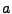Next: The Scale Factor Equation Up: Equations Previous: Selecting the Rescaling Variables

# Scale Factor Evolution

The Einstein equations for an FRW universe determine the evolution of the scale factor. In section 6.2.1 we derive the particular combination of these equations used by the program and then convert this equation to program variables. There is a difficulty that arises in solving this equation using the staggered leapfrog algorithm employed by LATTICEEASY, though, because the equation contains first derivative terms. This problem is explained in detail in section 6.4. Section 6.2.2 presents a revised formula that solves the problem. Finally, section 6.2.3 derives the equations used for fixed power-law expansion.

Subsections